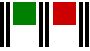What is a win/loss ratio?In a nutshell, the win/loss ratio is the average \$ size of a winning trade, divided by the average \$ size of a losing trade.

Suppose your system made 30 trades in a period of time and that there were 7 winning trades that made a total net profit of \$8,400. Suppose also there were 23 losing trades that made a total net loss of \$4,600.

The average winning trade works out at \$8,400 divided by 7 = \$1,200
and the average losing trade comes to \$4,600 divided by 23 = \$200.

The win/loss ratio is therefore \$1,200 divided by \$200 = 6.0

What use is the win/loss ratio?

Commodity traders are advised to run their winners and cut their losing trades, so the win/loss ratio is a reflection of how well they have achieved that aim.

The number of losing trades expected from a system invariably exceeds the number of winning trades because most of the market entry signals generated by the system will prove to be ‘incorrect’.

Commodity traders know that it is vital to allow their ‘small number’ of winning trades every opportunity to produce the maximum profit - whilst cutting their losing trades as soon as they go beyond their ‘risk limit’.

The more effective this strategy is, of course the greater the win/loss ratio will be.

The win/loss ratio is also used in combination with the win probability % to determine what is known as the ‘positive expectation’ of a system. The win probability is simply the % probability that a trade will be a winner - in this example it is 7 trades out of a total of 30 trades, which works out at 23%.Contact DavidDavid Bromley helps
new and aspiring systems